# PowerBI筛选器函数_ALL、ALLEXCEPT、ALLSELECTED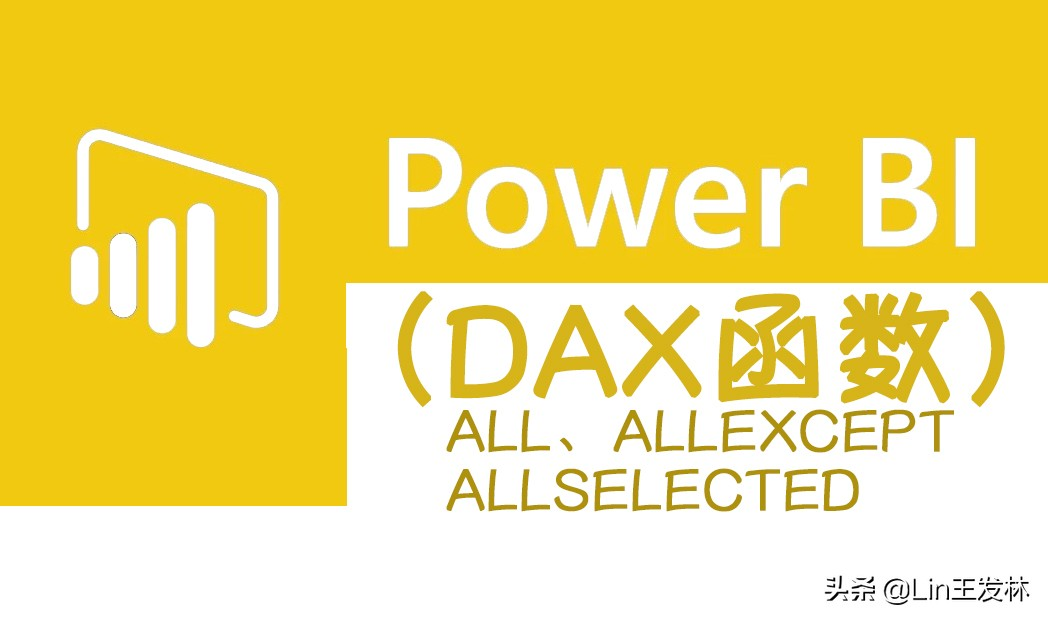【ALL】

``ALL([<table> | <column>[, <column>[, <column>[,…]]]])``

``销售金额(万) = SUM([总金额])/10000``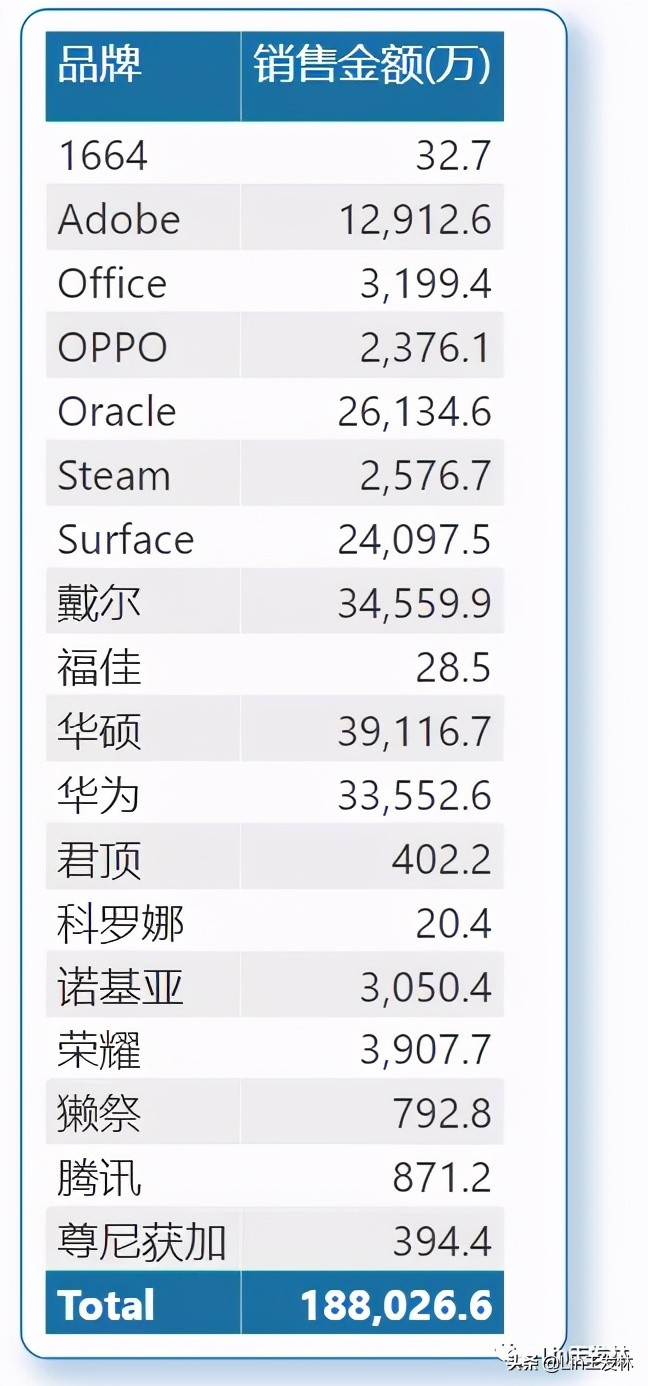``ALL销售金额 = CALCULATE([销售金额(万)],ALL('产品表'))``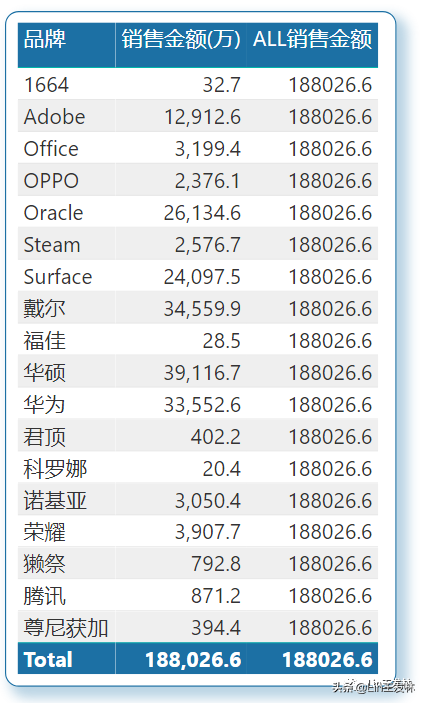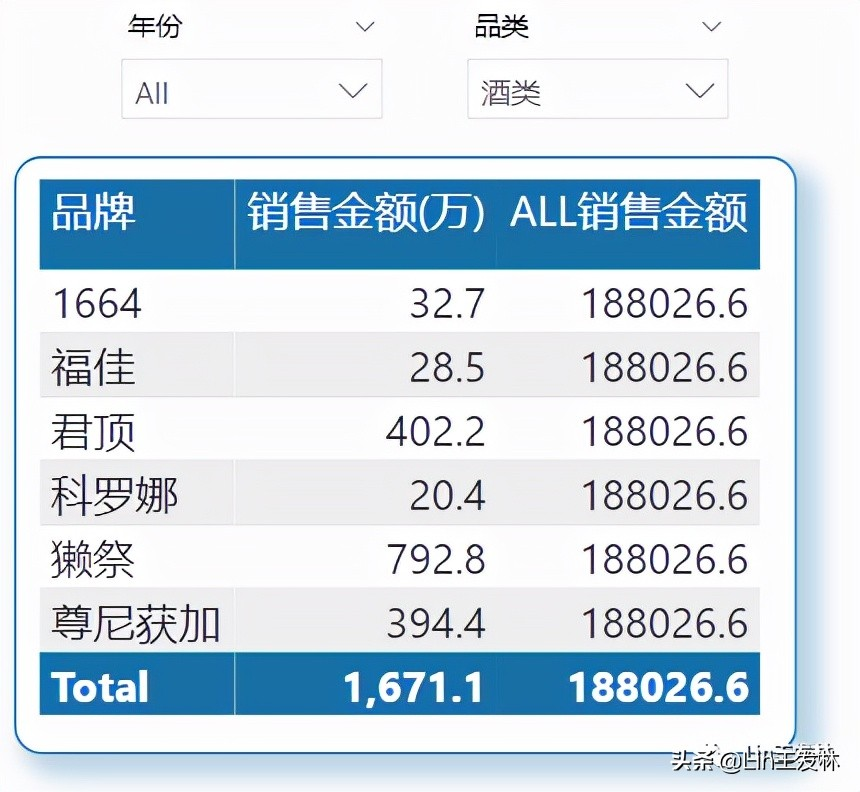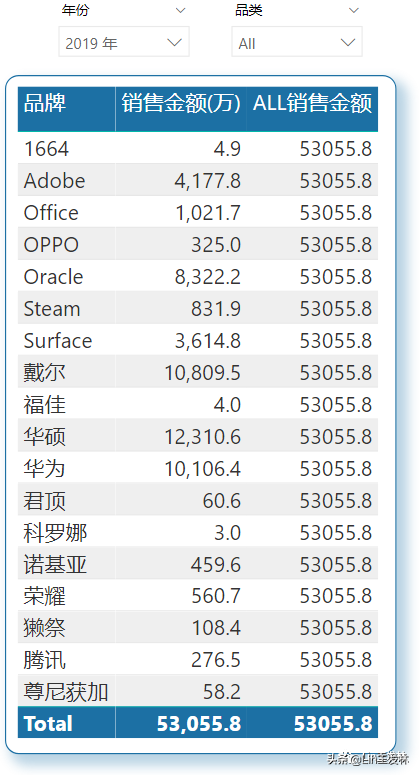``ALL销售金额2 = CALCULATE([销售金额(万)],ALL('产品表'),ALL('日期表'))``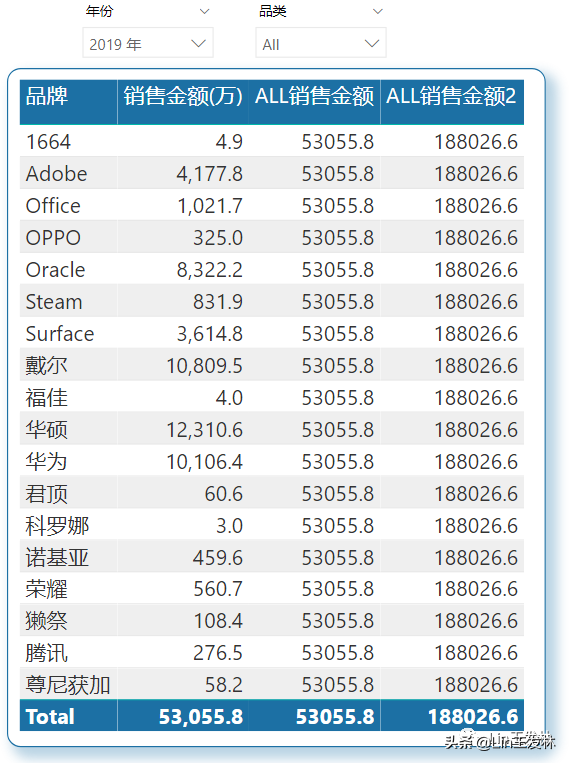``产品金额占比 = DIVIDE([销售金额(万)],[ALL销售金额])``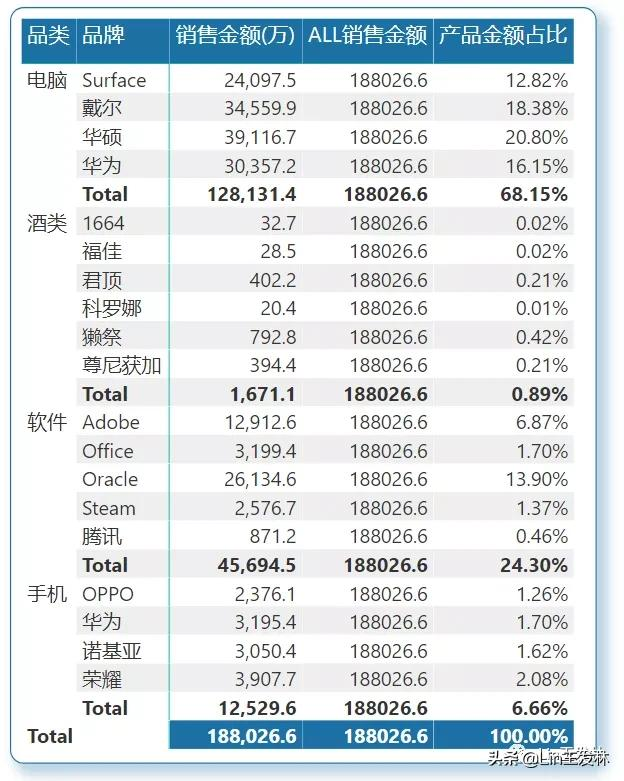【ALLEXCEPT】

``ALLEXCEPT(<table>,<column>[,<column>[,…]])``

ALLEXCEPT作用是除了指定的列之外，其他列全部清楚筛选器。第一个参数必须是对基表的引用，所有后续参数必须是对基列的引。下面就是我们要实现的品牌在每个品类中的占比计算金额↓

``ALLEXCEPT金额 = CALCULATE(    [销售金额(万)],    ALLEXCEPT('产品表','产品表'[品类]))````品类产品金额占比 = DIVIDE([销售金额(万)],[ALLEXCEPT金额])``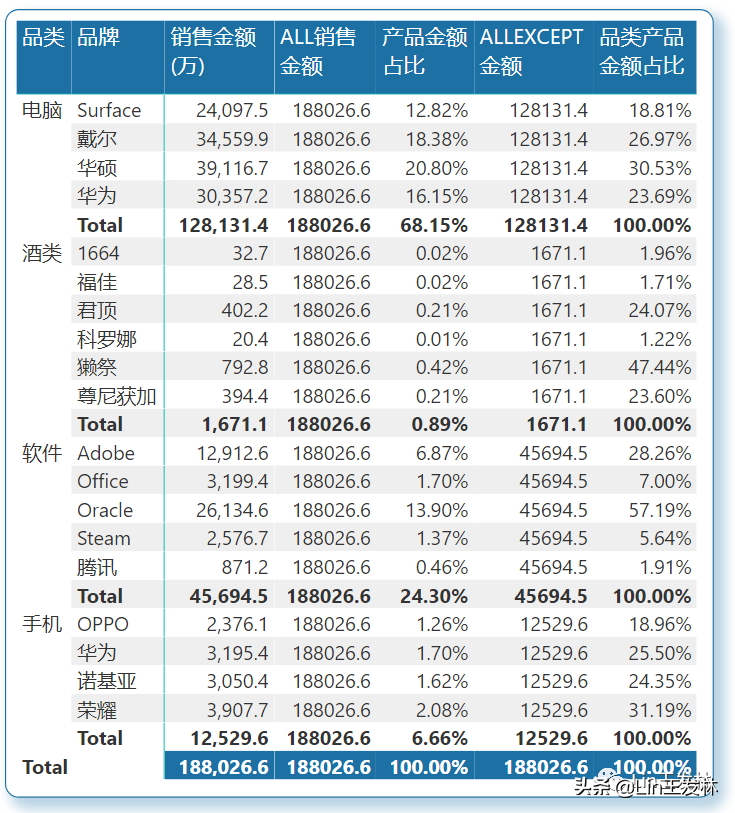【ALLSELECTED】

ALLSELECTED函数作用是删除当前查询的列和行中的上下文筛选器，同时保留所有其他上下文筛选器或显式筛选器。解释一下就是我们自己可以先根据切片器就行筛选，然后ALLSELECTED函数只作用于我们筛选后的表格，下面演示一下，我们只保留软件和手机两类。

``ALLSELECT金额 = CALCULATE([销售金额(万)],ALLSELECTED('产品表'))筛选金额占比 = DIVIDE([销售金额(万)],[ALLSELECT金额])``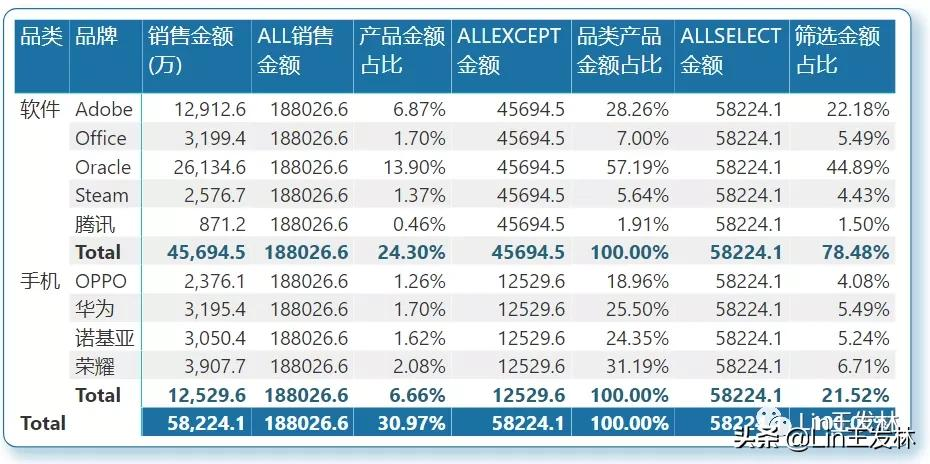End